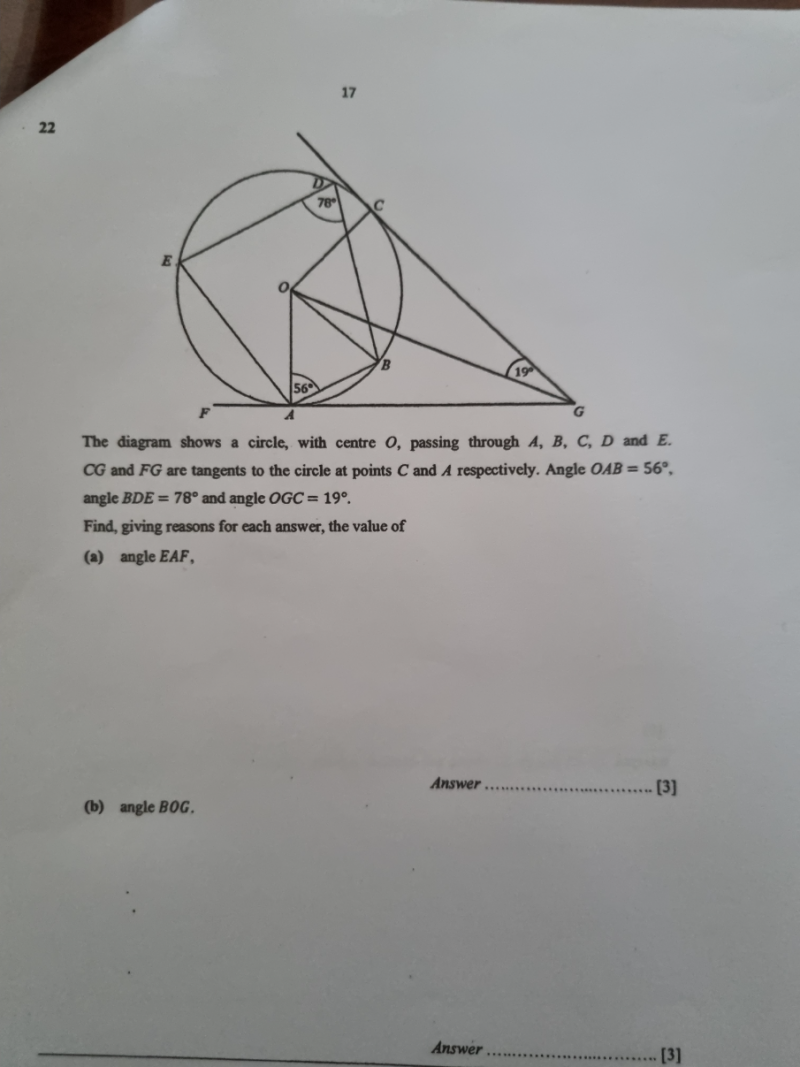# QuestionThank you

(a)  Angle EAB = 180 – 78 = 102 (opp angles in a circle quad add to 180)

Angle OAE = 102-56 =46

Angle OAF = 90 (line OA tangent to circle)

Hence Angle EAF = 90-46 =440

(b) CG= AG

Angle OAG = 90

Angle AOG = 180 – 90 – 19 = 71 (angles in a triangle)

Angle AOB = 180 -56 – 56 = 68 (angles in an isoceles triangle)

Hence Angle BOG = 71-68 = 30

0 Replies 1 Like ✔Accepted Answer